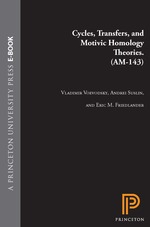# Cycles, Transfers, and Motivic Homology Theories. (AM-143)

Andrei Suslin
Eric M. Friedlander
Pages: 256
https://www.jstor.org/stable/j.ctt7tcnh

1. Front Matter
(pp. i-iv)
(pp. v-2)
3. 1 Introduction
(pp. 3-9)
Eric M. Friedlander, A. Suslin and V. Voevodsky

Our original goal which finally led to this volume was the construction of “motivic cohomology theory,” whose existence was conjectured by A.

Beilinson and S. Lichtenbaum (, , , ). Even though this would seem to be achieved at the end of the third paper, our motivation evolved into a quest for a deeper understanding of various properties of algebraic cycles. Thus, several of the papers presented here do not deal directly with motivic cohomology but rather with basic questions about algebraic cycles.

In this introduction, we shall begin with a short reminder of A. Beilinson’s formulation of motivic cohomology...

4. 2 Relative Cycles and Chow Sheaves
(pp. 10-86)

LetXbe a scheme. A cycle onXis a formal linear combination of points of the Zariski topological space ofX.A cycle is called an effective cycle if all points appear in it with non negative coefficients. Suppose thatXis a projective scheme over a fieldkof characteristic zero. Then for any projective embedding$i:X \to {{\bf{P}}^n}$the classical construction produces a projective variety${C_r}(X,i)$called the Chow variety of effective cycles of dimensionronXsuch thatk-valued points of${C_r}(X,i)$are in natural bijection with effective cycles of dimensionronX.Moreover, for...

5. 3 Cohomological Theory of Presheaves with Transfers
(pp. 87-137)

Letkbe a field and$Sm/k$be the category of smooth schemes overk.In this paper we study contravariant functors from the category$Sm/k$to additive categories equipped withtransfer maps.More precisely we consider contravariant functors$F:{(Sm/k)^{op}} \to A$together with a family of morphisms${\phi _{{X {\left/ {} \right.} S}}}(Z):F(X) \to F(S)$given for any smooth curve$X \to S$

over a smooth schemeSoverkand a relative divisorZonXoverSwhich is finite overS.If these maps satisfy some natural properties (see definition 3.1) such a collection of data is called apretheory over k.Some examples of pretheories are...

6. 4 Bivariant Cycle Cohomology
(pp. 138-187)
Eric M. Friedlander and Vladimir Voevodsky

The precursor of our bivariant cycle cohomology theory is the graded Chow group${A_*}(X)$of rational equivalence classes of algebraic cycles on a schemeXover a fieldk.In , S. Bloch introduced the higher Chow groups

$CH*(X,*)$in order to extend to higher algebraic K-theory the relation established by A. Grothendieck between${K_0}(X)$and${A_*}(X)$. More recently, Lawson homology theory for complex algebraic varieties has been developed (cf. , ), in which the role of rational equivalence is replaced by algebraic equivalence, and a bivariant extension$L*H*(Y,X)$ has been introduced. This more topological approach suggested a duality...

7. 5 Triangulated Categories of Motives Over a Field
(pp. 188-238)
In this paper we construct for any perfect fieldka triangulated category$D{M^{eff}}(k)$which is called thetriangulated category of (effective) motivic complexescomplexesoverk(the minus sign indicates that we consider only complexes bounded from the above). This construction provides a natural categorical framework to study different algebraic cycle cohomology theories (,,,) in the same way as the derived category of the etale sheaves provides a categorical framework for the etale cohomology. The first section of the paper may be considered as a long introduction. In §2.1 we give an elementary construction of a triangulated category$DM_{gm}^{eff}(k)$...